# Verbal Reasoning - Character Puzzles

### Exercise :: Character Puzzles - Character Puzzles 1

21.

Which one will replace the question mark ?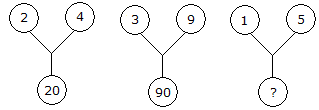A. 75 B. 26 C. 25 D. 20

Explanation:

(2)2 + (4)2 = 20

and (3)2 + (9)2 = 90

Therefore (1)2 + (5)2 = 26.

22.

Which one will replace the question mark ?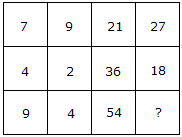A. 18 B. 24 C. 36 D. 58

Explanation:

(7 x 3) = 21 and (9 x 3) = 27

and (4 x 9) = 36 and (2 x 9) = 18

Therefore (9 x 6) = 54 and (4 x 6) = 24.

23.

Which one will replace the question mark ?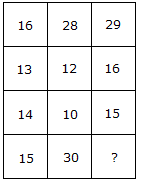A. 60 B. 30 C. 20 D. 45

Explanation:

(16 + 13) = (14 + 15)

and (28 + 12) = (10 + 30)

Therefore (29 + 16) = (15 + 30).

24.

Which one will replace the question mark ?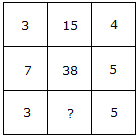A. 15 B. 19 C. 20 D. 18

Explanation:

(3 x 4) + 3 = 15

and (7 x 5) + 3 = 38

Therefore (3 x 5) + 3 = 18.

25.

Which one will replace the question mark ?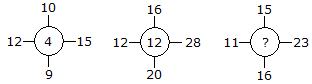A. 11 B. 14 C. 10 D. 12

Explanation:

(15 - 12) + (10 - 9) = 4

(28 - 12) + (16 - 20) = 12

Similarly, (23 -11) + (15- 16) = 11.